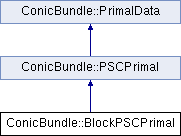ConicBundle
ConicBundle::BlockPSCPrimal Class Reference

implements a block diagonal PSCPrimal consisting of several PSCPrimal blocks More...

`#include <PSCPrimal.hxx>`

Inheritance diagram for ConicBundle::BlockPSCPrimal:## Public Member Functions

BlockPSCPrimal (const BlockPSCPrimal &pr, double factor=1.)
copy constructor

BlockPSCPrimal (const CH_Matrix_Classes::Indexmatrix &Xd, const std::map< CH_Matrix_Classes::Integer, PSCPrimal *> &pr, double factor=1.)

virtual PrimalDataclone_primal_data () const
returns a newly generated identical Object

virtual int assign_Gram_matrix (const CH_Matrix_Classes::Matrix &P)
for each element aij in the support set aij=<P.row(i),P.row(j)> More...

virtual int aggregate_primal_data (const PrimalData &it, double factor=1.)

virtual int aggregate_Gram_matrix (const CH_Matrix_Classes::Matrix &P, double factor=1.)
add factor*P*P^T to this More...

CH_Matrix_Classes::Integer get_nblocks () const
returns the number of blocks

CH_Matrix_Classes::Integer blockdim (CH_Matrix_Classes::Integer i) const
returns the size of block i

PSCPrimalblock (CH_Matrix_Classes::Integer i) const
returns the PSCPrimal of block i if there is one, 0 otherwise

virtual int scale_primal_data (double factor)
multiply/scale *this with a nonnegative factor

virtual int primal_ip (CH_Matrix_Classes::Real &value, const SparseCoeffmatMatrix &A, CH_Matrix_Classes::Integer column) const
if compatible evaluate value=ip(*this,A.column[i])

## Private Attributes

CH_Matrix_Classes::Indexmatrix Xdim
stores the sizes of the block

std::map< CH_Matrix_Classes::Integer, PSCPrimal * > primal
holds the PSCPrimal of blcok i if there is one

## Detailed Description

implements a block diagonal PSCPrimal consisting of several PSCPrimal blocks

The partition is generated on construction and at this point the various kinds of PSCPrimal variants are also assigned to each block by a map. For some blocks the assignment may be empty so that no information is aggregated for these. Note, the partition need not match some natural partition of the original coefficient data, even if it may and will in most practical applications.

## ◆ BlockPSCPrimal()

 ConicBundle::BlockPSCPrimal::BlockPSCPrimal ( const CH_Matrix_Classes::Indexmatrix & Xd, const std::map< CH_Matrix_Classes::Integer, PSCPrimal *> & pr, double factor = `1.` )

in this version control over the objects pointed to in pr is passed to this. this will delete them upon its destruction

## ◆ aggregate_Gram_matrix()

 virtual int ConicBundle::BlockPSCPrimal::aggregate_Gram_matrix ( const CH_Matrix_Classes::Matrix & P, double factor = `1.` )
virtual

add factor*P*P^T to this

The rows of P corresponding to each block are passed on the with a corresponding call to the PSCPrimal of this block

Implements ConicBundle::PSCPrimal.

## ◆ aggregate_primal_data()

 virtual int ConicBundle::BlockPSCPrimal::aggregate_primal_data ( const PrimalData & it, double factor = `1.` )
virtual

add factor*it to this (it must also be a BlockPSCPrimal with the same block partition)

Implements ConicBundle::PSCPrimal.

## ◆ assign_Gram_matrix()

 virtual int ConicBundle::BlockPSCPrimal::assign_Gram_matrix ( const CH_Matrix_Classes::Matrix & P )
virtual

for each element aij in the support set aij=<P.row(i),P.row(j)>

The rows of P corresponding to each block are passed on the with a corresponding call to the PSCPrimal of this block

Implements ConicBundle::PSCPrimal.

The documentation for this class was generated from the following file: